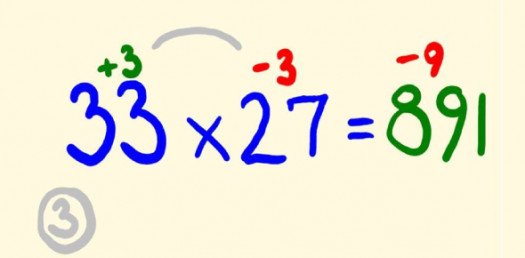# Multiply In 30 Seconds! Math Trivia Challenge Quiz

10 Questions | Attempts: 218SettingsDo you consider yourself a mathematics wiz with the power to complete multiplication questions in less than 30 seconds? Try this maths trivia challenge quiz to check it now. The quiz below is the first of many that challenges you to do just that, so if you are up to it, all you need to do is to press the start button. All the best, and share this quiz with others to check how easily you can multiply the two numbers without using a calculator.

• 1.
What is  5x125=?
• A.

125

• B.

145

• C.

625

• D.

25

• E.

155

• 2.
What is 5*8?
• A.

42

• B.

40

• C.

45

• D.

50

• 3.
What is 23*14?
• A.

322

• B.

222

• C.

422

• D.

522

• 4.
What is 90*90?
• A.

81

• B.

7900

• C.

810

• D.

8100

• 5.
Solve for 50*90.
• A.

450

• B.

540

• C.

4500

• D.

5000

• 6.
What is 0*0?
• A.

0

• B.

Infinite

• C.

1

• D.

None of the above

• 7.
What is 77x26=?
• A.

2002

• B.

2006

• C.

2008

• D.

2010

• E.

2099

• 8.
What is 5555x5555=?
• A.

47532155

• B.

85265445

• C.

85463278

• D.

30858025

• E.

74563285

• 9.
What is 8989x9999=?
• A.

96836363

• B.

89881011

• C.

41256325

• D.

78968965

• E.

00012354

• 10.
What is 5698x1235=?
• A.

7037039

• B.

7037030

• C.

7023698

• D.

7032547

## Related TopicsBack to top
×

Wait!
Here's an interesting quiz for you.